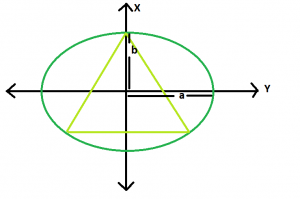GeeksforGeeks App
Open AppBrowser
Continue

# Largest triangle that can be inscribed in an ellipse

Given an ellipse, with major axis length 2a & 2b, the task is to find the area of the largest triangle that can be inscribed in it.
Examples:

```Input: a = 4, b = 2
Output: 10.3923

Input: a = 5, b = 3
Output: 10.8253```Approach: So we know the ellipse is just the scaled shadow of a circle.Let’s find the scaling factor.

x^2/a^2 + y^2/b^2 = 1 is an ellipse. Rewrite this as:
(y*(a/b))^2+x^2 = a^2

This is just a vertically scaled down circle of radius a (think light falls from the top at an angle), and the vertical factor is a/b. The biggest triangle in the ellipse is then a scaled up version of the biggest triangle in the circle. Using a little geometry and taking symmetry into account, we can understand that the biggest such triangle is the equilateral one. It’s sides will be √3a and the area will be (3√3)a^2/4
Translating this to ellipse terms – we scale the horizontal dimension up by a factor a/b, and the area of the biggest triangle in the ellipse is,

A = (3√3)a^2/4b

Below is the implementation of above approach:

## C++

 `// C++ Program to find the biggest triangle``// which can be inscribed within the ellipse``#include ``using` `namespace` `std;` `// Function to find the area``// of the triangle``float` `trianglearea(``float` `a, ``float` `b)``{` `    ``// a and b cannot be negative``    ``if` `(a < 0 || b < 0)``        ``return` `-1;` `    ``// area of the triangle``    ``float` `area = (3 * ``sqrt``(3) * ``pow``(a, 2)) / (4 * b);` `    ``return` `area;``}` `// Driver code``int` `main()``{``    ``float` `a = 4, b = 2;``    ``cout << trianglearea(a, b) << endl;` `    ``return` `0;``}`

## Java

 `//Java Program to find the biggest triangle``//which can be inscribed within the ellipse` `public` `class` `GFG {` `    ``//Function to find the area``    ``//of the triangle``    ``static` `float` `trianglearea(``float` `a, ``float` `b)``    ``{` `     ``// a and b cannot be negative``     ``if` `(a < ``0` `|| b < ``0``)``         ``return` `-``1``;` `     ``// area of the triangle``     ``float` `area = (``float``)(``3` `* Math.sqrt(``3``) * Math.pow(a, ``2``)) / (``4` `* b);` `     ``return` `area;``    ``}` `    ``//Driver code``    ``public` `static` `void` `main(String[] args) {``    ` `        ``float` `a = ``4``, b = ``2``;``         ``System.out.println(trianglearea(a, b));``    ``}``}`

## Python3

 `# Python 3 Program to find the biggest triangle``# which can be inscribed within the ellipse` `from` `math ``import` `*` `# Function to find the area``# of the triangle``def` `trianglearea(a, b) :` `    ``# a and b cannot be negative``    ``if` `a < ``0` `or` `b < ``0` `:``        ``return` `-``1` `    ``# area of the triangle``    ``area ``=` `(``3` `*` `sqrt(``3``) ``*` `pow``(a, ``2``)) ``/` `(``4` `*` `b)` `    ``return` `area`  `# Driver Code``if` `__name__ ``=``=` `"__main__"` `:` `    ``a, b ``=` `4``, ``2``    ``print``(``round``(trianglearea(a, b),``4``))`  `# This code is contributed by ANKITRAI1`

## C#

 `// C# Program to find the biggest``// triangle which can be inscribed``// within the ellipse``using` `System;` `class` `GFG``{` `// Function to find the area``// of the triangle``static` `float` `trianglearea(``float` `a, ``float` `b)``{` `// a and b cannot be negative``if` `(a < 0 || b < 0)``    ``return` `-1;` `// area of the triangle``float` `area = (``float``)(3 * Math.Sqrt(3) *``                         ``Math.Pow(a, 2)) / (4 * b);` `return` `area;``}` `// Driver code``public` `static` `void` `Main()``{``    ``float` `a = 4, b = 2;``    ``Console.WriteLine(trianglearea(a, b));``}``}` `// This code is contributed``// by Akanksha Rai(Abby_akku)`

## PHP

 ``

## Javascript

 ``

Output:

`10.3923`

Time Complexity: O(1)

Auxiliary Space: O(1)

My Personal Notes arrow_drop_up